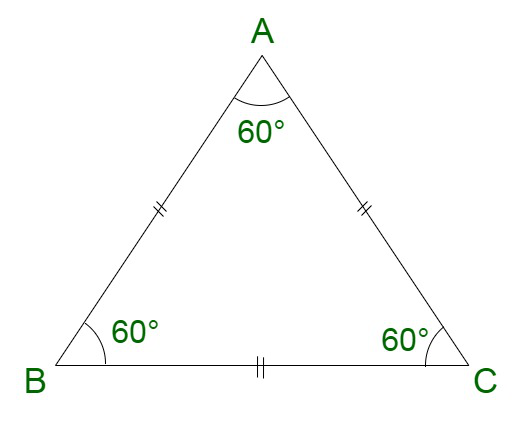GeeksforGeeks App
Open AppBrowser
Continue

# How to find the perimeter of a square and an equilateral triangle?

Square is a geometrical figure which is formed of four equal sides. It is a regular quadrilateral where all the angles are right angles. Basically, it can be considered as a special case of a rectangle, where all the sides are equal in length. For instance, a square courtyard.

Properties of square

• All four sides of a square are equal.
• The diagonals of a square are equal.
• All the four angles of a square are equal.
• The diagonals of a square bisect its angles.

### Perimeter of a square

The perimeter of a square is referred to as the total length of the boundary enclosing the geometrical figure.

Perimeter of square = Sum of all sides of a square

Let us assume s to be the side of the square.

Since, we know, all the sides of the square are equal.

Perimeter of square = s + s + s + s

Perimeter of square = 4s ….. (I)

Example: Find the perimeter of the square with each side of 10 units.

Solution:

Here we have been given each side of square is 10 units

As we know that,

Perimeter of square, (P) = Side + Side + Side + Side

Perimeter of square, (P) = 10 + 10 + 10 + 10

Or

Perimeter of square, (P) = 4 × Side

Perimeter of square, (P) = 4 × 10

Therefore,

Perimeter of square, (P) = 40 units

### Equilateral Triangle

An equilateral triangle, also known as the equiangular triangle is a special case of a triangle, where all three sides are equal in length. These sides also subtend equal angles at the corners. The summation of the length of sides yields the total perimeter of the triangle.

Properties of Equilateral Triangle

An equilateral triangle is characterized by the following properties:

• All three sides are equal.
• All three angles are equal to 60°

In the figure given, the sides of the triangle ABC are equal, i.e., AB = BC = CA.

### Perimeter of Equilateral Triangle Formula

Let us assume a to be the side of the equilateral triangle.

Now,

Perimeter of equilateral triangle = Sum of all sides

= a + a + a

Now, the perimeter of an equilateral triangle = 3a### Sample Questions

Question 1. Calculate the perimeter of the square, with a diagonal of 20 units.

Solution:

Here we have to calculate the perimeter of the square using the given diagonal dimension,

To find the perimeter of the square using diagonal length use the formula below,

Perimeter of square = 4 × √2 ()

Here diagonal = 20 units

By applying value of diagonal in the above formula

Perimeter of square =Perimeter of square = 40√2

Question 2. Calculate the perimeter of the square if its area is 64 m2?

Solution:

Here we have to calculate the perimeter of the square using the given area,

To find the perimeter of the square using the area of square use the formula below,

Perimeter of square =Here Area = 64 m2

By applying the value of the area in the above formula

Perimeter =  4 × √64

Perimeter of square = 4 × 8

Perimeter of square = 32 m

Question 3. Assume that the area of an equilateral triangle is 64√3 cm2, find its perimeter?

Solution:

Here we have to find the perimeter of equilateral triangle using its area

Formula for equilateral triangle area =Area of equilateral triangle = 64√3

64√3 =a2a = √256

a = 16

Therefore,

Side of the equilateral triangle is 16 cm

Now further finding perimeter of equilateral triangle

Perimeter of equilateral triangle = side + side + side = 3a

Perimeter of equilateral triangle = 3 × 16

Perimeter of equilateral triangle = 48 cm

Question 4. Calculate the perimeter of an Equilateral triangle in height of the triangle is 16√3 cm.

Solution:

Here we have to find the Perimeter of the equilateral triangle with the height 16√3 cm

Formula for calculating perimeter using height is given below

Height =Here a is the side of the equilateral triangle

16√3 =a =a = 32 cm

Now further finding perimeter of the equilateral triangle

Perimeter of equilateral triangle = side + side + side = 3a

Perimeter of equilateral triangle = 3 × 32

Perimeter of equilateral triangle = 96 cm

Question 5. If the perimeter of an equilateral triangle and square are the same and the side of an equilateral triangle is 15 cm, then find the perimeter of the square and its side?

Solution:

Here we have to find the perimeter of the square using the given side of an equilateral triangle and we are given that the perimeter of the square and triangle are the same

First, we will find the perimeter of the equilateral triangle

Perimeter of equilateral triangle = side + side + side = 3a

The perimeter of equilateral triangle = 3 × 15

The perimeter of equilateral triangle = 45 cm

According to the question

The perimeter of equilateral triangle = Perimeter of a square

Therefore,

Perimeter of square = 45 cm

Perimeter of square = side + side + side + side = 4 × side = 45 cm

4 × side = 45 cm

Side =Side = 11.25 cm

My Personal Notes arrow_drop_up
Related Tutorials